## Improving path¶

• Sequence of adjacent networks:

• Link is added if it benefits both agents, at least one strictly
• Link is deleted if either agent benefits from its deletion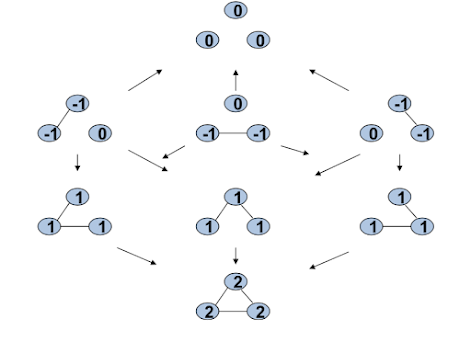## Two PNS networks¶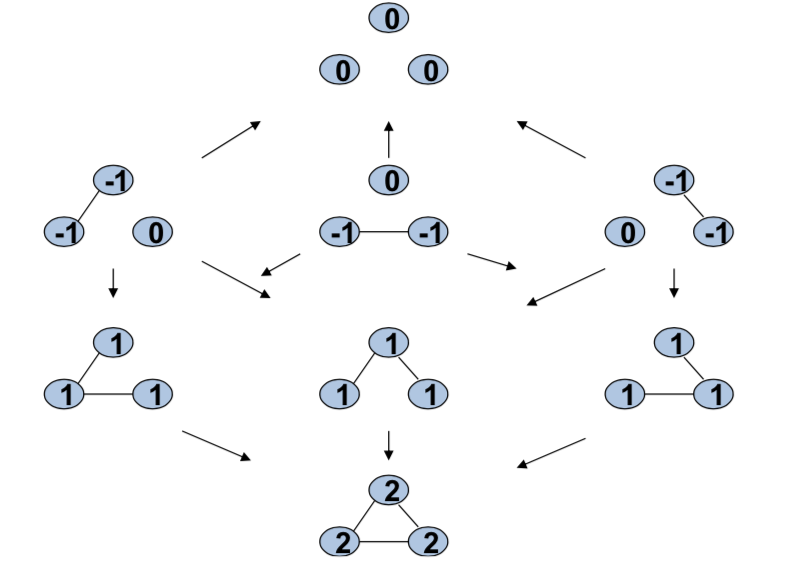## Stochastic Stability¶

• Add trembles/errors to improving paths
• Start at some network and with equal probability on all links choose a link:

• Add that link if it is not present and both agents prefer to add it(at least one strictly)
• delete that link if it is present and one of the two agents prefers to delete it
• Reverse the above decision with probability $\epsilon >0$
• Trembles/errors: $\epsilon >0$

• Finite state, irreducible, aperiodic Markov chain

• You can get from any network to any other network through a series of errors and improvments. There's a finite set of possible networks you could be in, it's irreducible, in the sense that from anyone you can get to any other one, and it's aperiodic. You could move in odd cycles, even cycles, so this is a very nicely behaved Markov chain. It's going to have a nice distribution over how much time you'll spend at each. So, pro, given epsilon and we'll have an idea of how much time you would spend at each network in this process over time. There's a steady state distribution which gives us the long-run distribution of how much time you'll spend at each one of these networks.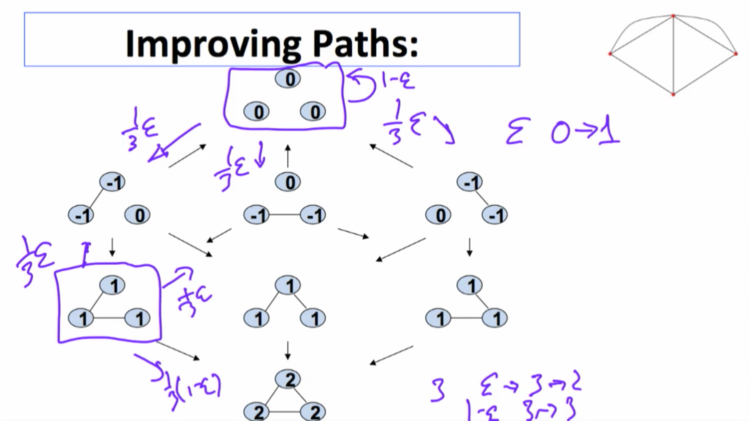So, what's going to happen is, if we recognize a link, they don't, it doesn't want to be added but with a probability epsilon it would be added. So, there's a probability of one third epsilon that you would end up recognizing this link and adding it by accident. There's a probability one third epsilon that you would move here. And a probability one third epsilon that you would move in this direction. So, from a network with zero links there is a probability of epsilon that you go from zero links to one link. And there's a probability of one minus epsilon that you'd just stay there. Okay? And, and so forth. If we're here with two, two, with three links. Then there's a probability epsilon that you go from three to two and the probability of one minus epsilon that you go from three back to three. And so forth, okay. And so from, from one of these networks there's a one third times one minus epsilon chance that you go to the complete network. There's a one third chance times epsilon you go and delete a link that you wanted. one third chance times epsilon here and so forth. So, for each one of these things we can figure out what's the probability that you move from a network with zero links to one with one. From one with one, to two, and so forth. So instead of keeping track of all of the networks, let's just keep track of them in terms of how many links they have.

# Associated Markov Chain¶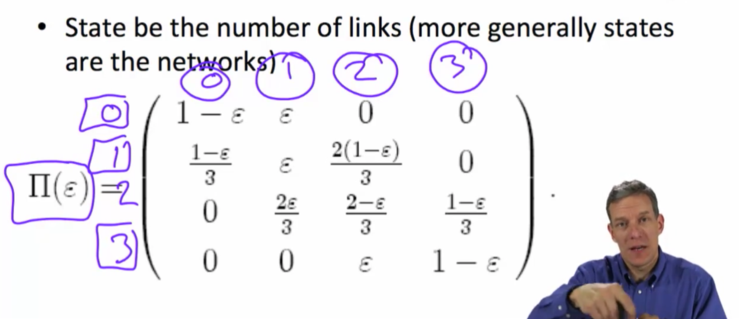That's a well defined Markov chain. And, given this, you can figure out what's the probability that you're going to stay in every state. So there's a solution of how much time you spend in state zero, how much time will you spend in state one and state two and state three. So what's the average frequency of the number of periods you're going to spend in each one of these things? That's a well defined Markov chain problem. You look for the steady state distribution. In this case it happens to be the left hand side unit eigenvector of this matrix. If you don't remember things on Markov chains or if you haven't seen Markov chains before you can find detailed things on on the internet. Also, I have some pages in my book that just goes through the basics of Markov chains. There is lots of sources where you can find information about this. the important thing is that once we've got this process, it's a well defined dynamic process.

And we can figure out how much time you are going to spend in each different state. In this case when you look at the, the probability, that, the fraction of time you're going to spend in zero link network, in a one link network, in a two link network and in a three link network, this is the answer to that unique steady state distribution. And you can find this by just checking what the left hand side unit eigenvector of that matrix was.

# Unique steady state distribution¶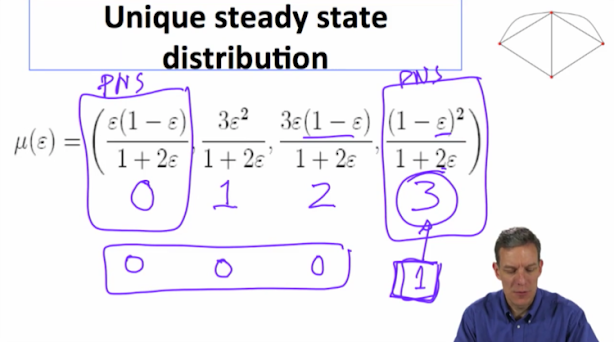So what's the interesting thing about this? Well the interesting thing about this. Well, the interesting thing about this was we had two Pairwise Stable Nash Networks. The empty network and the complete network. And if you look at the amount of time you're spending in each one of these things, as epsilon gets very, very small, right, as epsilon goes to zero, this approaches one.

This term ex, approaches zero. This term approaches zero and this term approaches zero. So, this process is going to spend more and more of its time in this three link network over time. So, even though both of these were Pairwise Nash Stable. This dynamic process is going to end up spending, as epsilon becomes very small, most of its time in the three length network. And so you can look at that by looking at the limit of this unique steady state distribution. This is what's known as stochastic stability in the game theory literature. It's a technique of, of refining equilibria that has become quite powerful. and Alison and I used this to, to, to develop methods of, of working through dynamics in, in networks. And here it consists of prediction of which network is going to be visited most often and stayed at for most of the time in this process as epsilon becomes quite small. And one thing to notice is that, as it's placing probability one here in the limit. That's not the same, as the steady states of the limit process. So if you actually look at the process that had zero errors in it, you could have ended up in either the empty network or the full network. So the steady states of the, of the limit process, where you have no noise, is different than the limit of the process with little bits of noise. So the little bits of noise here are very important in making predictions

Limit place probability 1 on the complete network, not the same as the steady states of limit process - which are any with probalility on both the ocmplete and empty

# Two PNS networks¶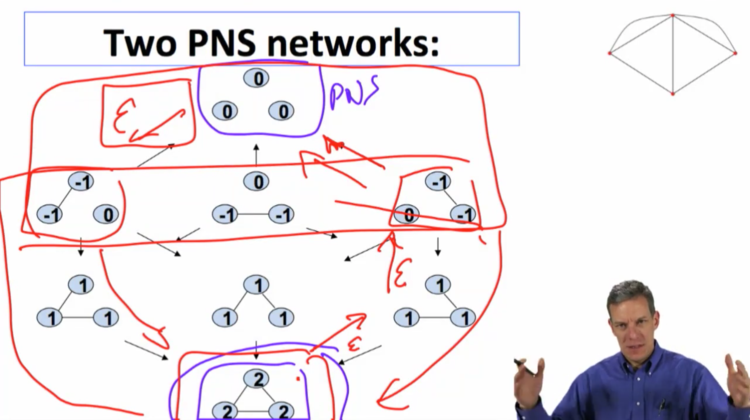So, if we suppose we're at at the network with three links. Well, we can make an error. If we make an error and bounce to one of these, so there's probably epsilon of that happening. most likely it's going to come back. You would need two errors in order to get to a point where you might get to to the other network, to the empty network, right? So you'd have to, you'd have to make two errors in a row before you could get to a point where you're going to find a network which then naturally sort of has a tendency to lead back to the empty network or the other Pairwise Nash Stable Network. In contrast, one error on this network leads you to something which then leads naturally to the, to this. So when you've got errors, it's much harder in some sense that this stochastic stability notion means this is a more stable, the full network is a more stable network. It's harder to get away from that network. And it's easier to, relatively easier, and it only takes one error to get away from the empty network. And so, what this does is, is it begins to look at what is known as basins of attraction. When you look at these dynamic processes, this one has a larger basin of attraction. If you get to any of these networks, you can get back to this one. To get to this one you really can only get there from, from a smaller set of states. And as, as you begin to look at this, this process more naturally gives you a complete network than a smaller one. So, this is a powerful tool for looking at dynamics. you can simulate these things so once, you know, this we can solve for explicitly with, with only three, three nodes. And relatively small number of, of networks. As you begin to enrich these kinds of processes, they're going to get more complicated, but you can begin to analyze them by simulation. So the

## Ideas¶

• More errors to leave basin of attraction of the complete network than to leave the basin of attraction of empty network

• More generally need to keep track of basins of attraction of many states (via a theorem of Friedlin and Wentzel (1984))

## Turn Game into Markov Chain¶

• Much is known about Markov chains

• Can leverage that to prove results

• Can be difficult to get analytic solutions in some contexts with large societies, but can simulate

## Modeling Stability¶

• Beyond Pairwise Stability - Allowing other deviations

• multiple links by individuals
• coordinated deviations
• Existence questions

• Dynamics

• Stochastic Stability

• Forward looking behavior

• Build transfers into the formation process